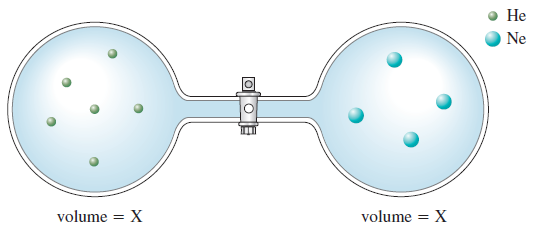Problem: Consider the flasks in the following diagrams.c. Solve for the final pressure in terms of the original pressures of helium and neon. Assume temperature is constant.

FREE Expert Solution
85% (234 ratings)
FREE Expert Solution

We’re being asked to solve for the final pressure in terms of the original pressures of helium and neon

Recall that the partial pressure of a gas (PGas) in a mixture is given by:

$\overline{){{\mathbf{P}}}_{{\mathbf{gas}}}{\mathbf{=}}{{\mathbf{\chi }}}_{{\mathbf{gas}}}{{\mathbf{P}}}_{{\mathbf{total}}}}$

where:

χGas = mole fraction of the gas

Ptotal = total pressure of the gas mixture

Mole fraction can be calculated using the equation shown below:

Calculate the mole fraction of each gas:

85% (234 ratings)
Problem Details

Consider the flasks in the following diagrams.c. Solve for the final pressure in terms of the original pressures of helium and neon. Assume temperature is constant.

What scientific concept do you need to know in order to solve this problem?

Our tutors have indicated that to solve this problem you will need to apply the Partial Pressure concept. You can view video lessons to learn Partial Pressure Or if you need more Partial Pressure practice, you can also practice Partial Pressure practice problems .

How long does this problem take to solve?

Our expert Chemistry tutor, Alisa took 2 minutes to solve this problem. You can follow their steps in the video explanation above.

What professor is this problem relevant for?

Based on our data, we think this problem is relevant for Professor Boesdorfer's class at ISU.

What textbook is this problem found in?

Our data indicates that this problem or a close variation was asked in . You can also practice practice problems .